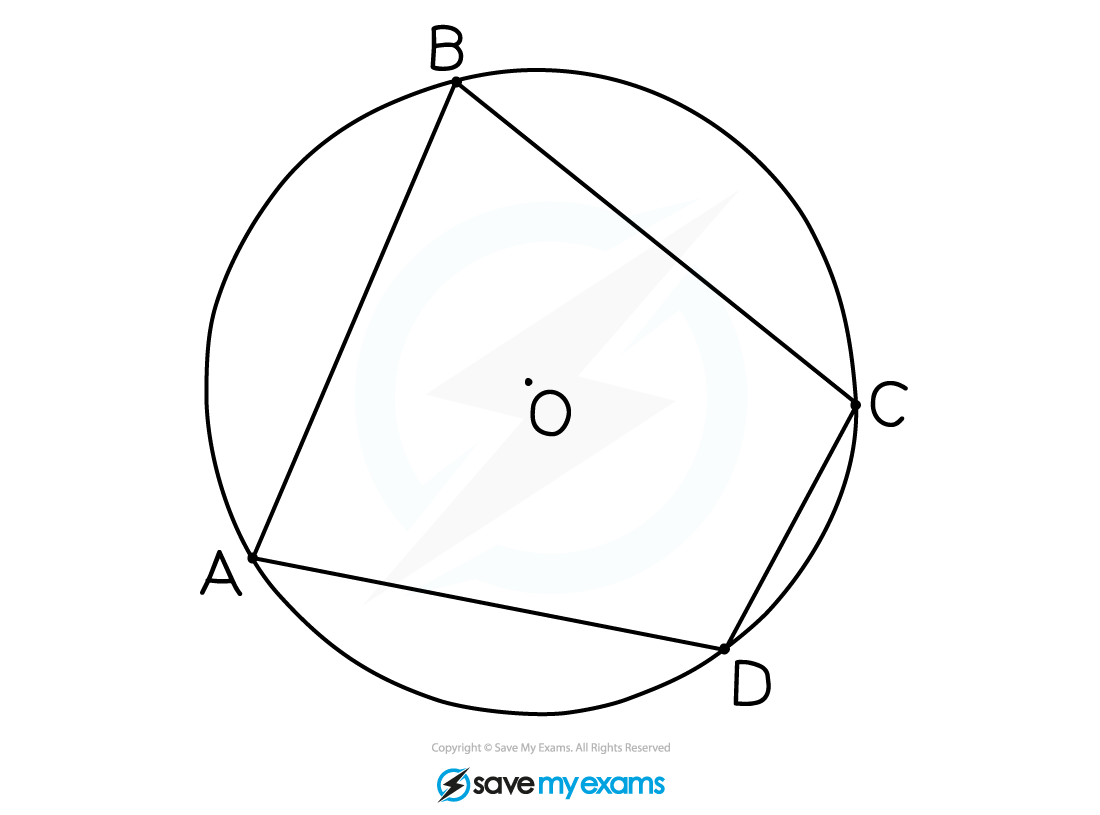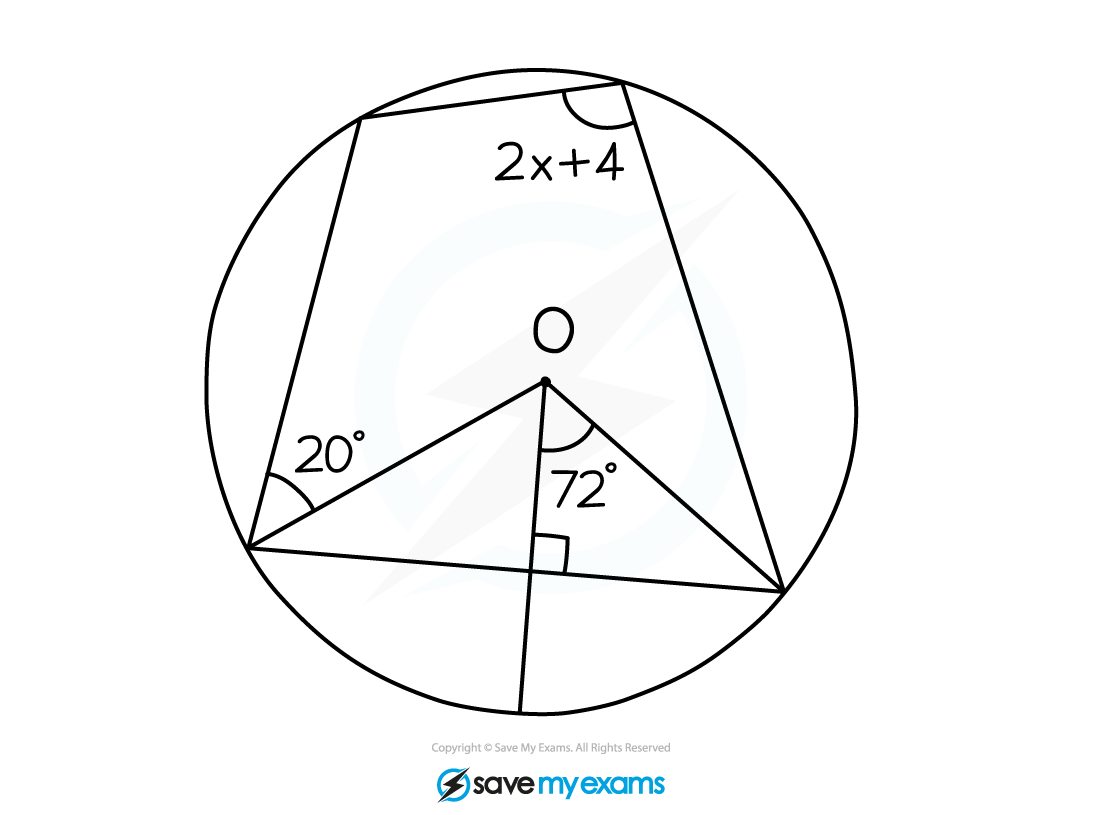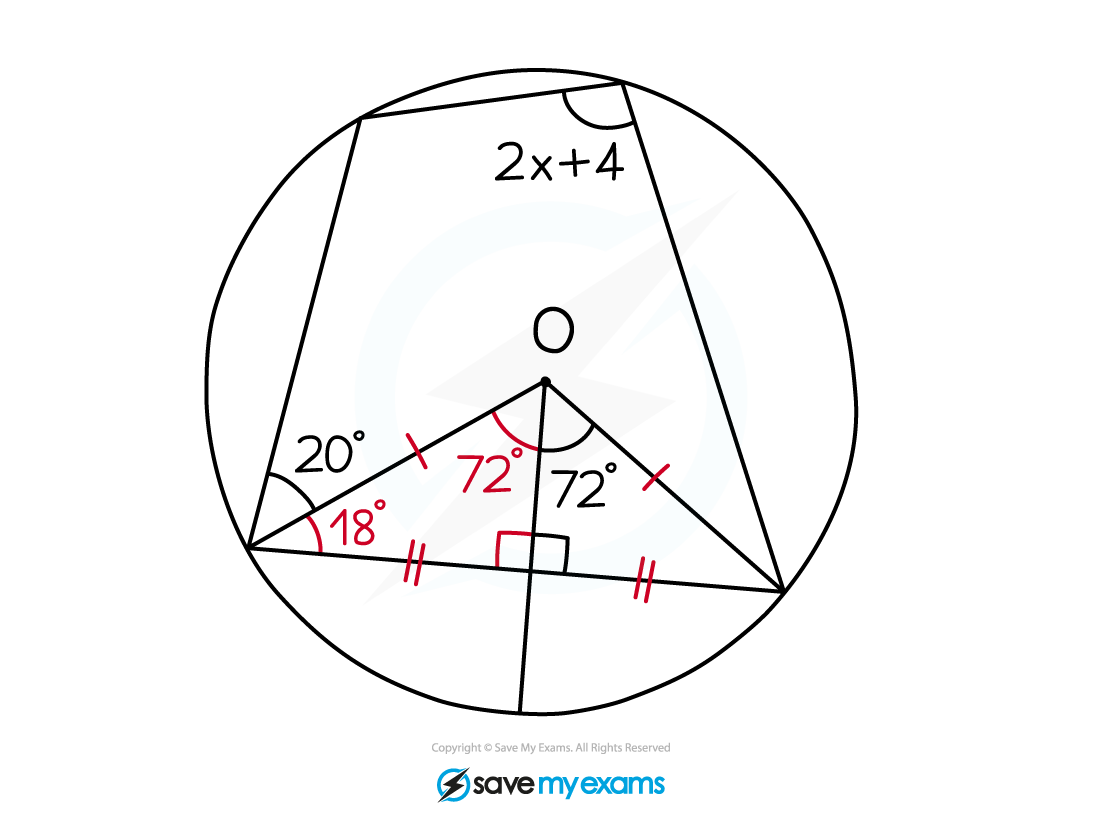# Edexcel IGCSE Maths 复习笔记 4.6.3 Circle Theorems - Cyclic Quadrilaterals

Edexcel IGCSE Maths 复习笔记 4.6.3 Circle Theorems - Cyclic Quadrilaterals• Double-check is that all 4 vertices of the quadrilateral are on the circumference
• The diagram below shows a common scenario that is NOT a cyclic quadrilateral:2. The perpendicular bisector of a chord is a radius• This is also easier to see than remember from its description
• Problems here involve the radii being joined to the end of the chords and so creating two congruent triangles

#### Exam Tip

Remember to add anything you can to a diagram you have been given – write in any angles and lengths you can work out, even if they don’t seem relevant to the actual question.

#### Worked Example#### Worked Example